# Weight and Balance Theory (Part 1)

## Weight and Balance Theory

Two elements are vital in the weight and balance considerations of an aircraft.
• The total weight of the aircraft must be no greater than the maximum weight allowed by the FAA for the make and model of the aircraft.
• The center of gravity (CG), or the point at which all of the weight of the aircraft is considered to be concentrated, must be maintained within the allowable range for the operational weight of the aircraft.

### Arm

The arm is usually measured and expressed in inches and refers to the horizontal distance between the CG of an item or object and the datum, a point from where all measurements are taken. Arms to the left of the datum are negative (–) and those to the right of the datum are positive (+). The datum is an imaginary vertical plane from which all horizontal distances are measured for balance purposes. The position of the reference datum varies by aircraft design and manufacturer. When the datum is located off of the lever and to the left, all of the arms are positive and computational errors are minimized. Note: When the datum is established ahead of the aircraft, for example at the aircraft nose, all of the arms are positive and computational errors are minimized.

### Moment

A moment is a force that tries to cause rotation and is the product of the arm, in inches, and the weight, in pounds. Moments are generally expressed in pound-inches (lb-in) and may be either positive or negative.

## The Law of the Lever

Weight and balance problems are based on the physical law of the lever. This law states that a lever is balanced when the weight on one side of the fulcrum (a pivot point for the lever) multiplied by its arm is equal to the weight on the opposite side multiplied by its arm. In other words, the lever is balanced when the sum of the moments about the fulcrum is zero. This is the condition in which the positive moments (those that try to rotate the lever clockwise) are equal to the negative moments (those that try to rotate it counterclockwise). In an aircraft, the balance point is referred to as the CG.

One of the easiest ways to understand weight and balance is to consider a lever with weights placed at various locations. The balance point or CG of the lever can be changed by either moving the weights closer or farther from the fulcrum or by increasing or decreasing the weights. The balance point or CG of a lever may be determined by using these four steps:
1. Measure the arm of each weight in inches from the datum.
2. Multiply each arm by its weight in pounds to determine the moment in pound-inches of each weight.
3. Determine the total of all weights and of all the moments. (Disregard the weight of the lever).
4. Divide the total moment by the total weight to determine the balance point.

Consider these facts about the lever in Figure 1. The 100-pound weight A is located 50 inches to the left of the fulcrum (the datum, in this instance), and it has a moment of100 × –50 = –5,000 lb-in. The 200-pound weight B is located 25 inches to the right of the fulcrum, and its moment is200 × +25 = +5,000 lb-in. In Figure 2, the sum of the moments is –5,000 + 5,000 = 0, and the lever is balanced. The forces that try to rotate it clockwise have the same magnitude as those that try to rotate it counterclockwise. If either weight is moved or changed, the balance point or CG changes and the lever becomes unbalanced.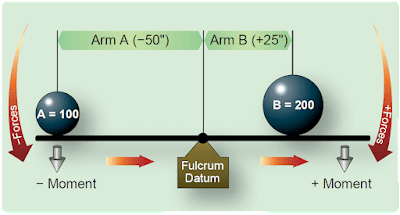Figure 1. Balance lever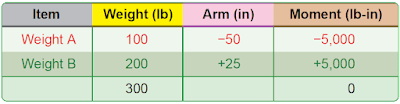Figure 2. Balance point locations

In Figure 3, the datum is located off the lever to the left of weight A. Using the information provided in Figure 3, determine the balance point by making a chart like the one in Figure 4.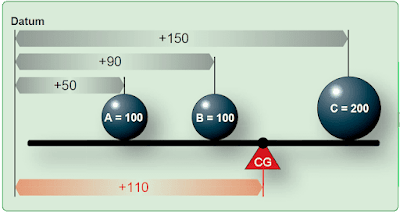Figure 3. Balance lever datum located off the lever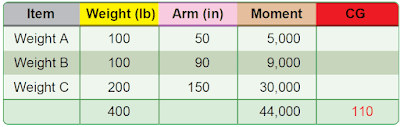Figure 4. Finding balance point with datum located off the lever

As noted in Figure 4, A weighs 100 pounds and is 50 inches from the datum; B weighs 100 pounds and is 90 inches from the datum; C weighs 200 pounds and is 150 inches from the datum. The total of the weights is 400 pounds, and the total moment is 44,000 lb-in.

Determine the balance point by dividing the total moment by the total weight. A balance point is equal to the CG and can be mathematically written as:

CG= total moment/total weight

To prove this is the correct balance, move the datum to a location 110 inches to the right of the original datum and determine the arm of each weight from this new datum. [Figure 5] Then, make a new chart similar to the one in Figure 6. If the balance point is correct, the sum of the moments is zero.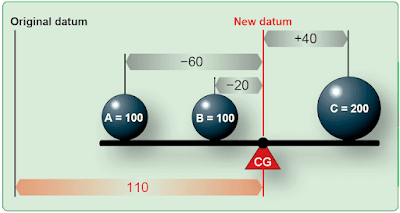Figure 5. Locating balance point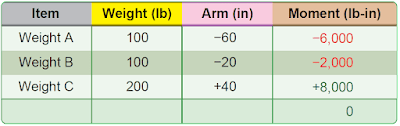Figure 6. Proving balance point with three weights is correct

The new arm of weight A is 60 inches (the difference between 110 and 50), and since this weight is to the left of the datum, its arm is negative or –60 inches. The new arm of weight B is 20 inches (110 – 90), and it is also to the left of the datum, so it is –20; the new arm of weight C is 40 inches (150 – 110). It is to the right of the datum and is therefore positive.

The lever is balanced when the sum of the moments is zero. The location of the datum used for determining the arms of the weights is not important; it may be in various locations, but all of the measurements must be made from the same datum location.

The procedure for finding the balance point is the same anywhere the datum is located. In Figure 7, the datum is located at C. Weight A has an arm of –100 inches (negative because it is to the left) of the datum and weight B has an arm of –60 inches from the datum. The table in Figure 8 is used to determine the new balance point.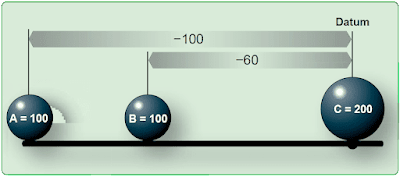Figure 7. Locating balance point with datum at C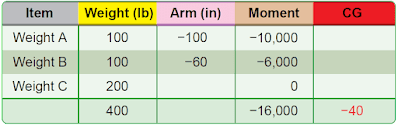Figure 8. Determining new balance point

To verify that this is the correct balance point, move the datum 40 inches to the left of the original datum and determine the arm of each weight from this new datum as in Figure 9.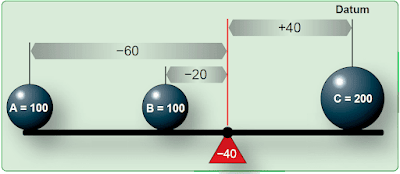Figure 9. Locating balance point with datum left of original

The new arm for weight A would be –100 + 40 = –60; for weight B, –60 + 40 = –20; and point C, is +40. The lever is balanced and the balance point is correct when the sum of the moments is zero. [Figure 10]